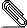# [NTG-context] Numbering in mathalignment not working

```Hi,

[I am on version 2022.05.11 11:36]```
```
I think either a bug might have crept into one of the recent uploads or there
is some change in behaviour – numbering of statements inside mathalignment
blocks is not working: only one statement is numbered even if multiple are
requested.

The following example does not produce the expected result (sample attached):
both the equations should be numbered when only the first one is –

\starttext

\placeformula
\startformula
\startmathalignment
\NC a \NC = b \NR[eq:one]
\NC c \NC = d \NR[eq:two]
\stopmathalignment
\stopformula

\stoptext

Also the [+] options and the second optional argument of \NR does not work as
expected. Has its behaviour changed?

I ran into this when I compiled a large maths-heavy document and while proof-
reading noticed numerous missing references to statements inside

Thank you,
Sreeram```align-numbering.pdf
```___________________________________________________________________________________